Navigation Panel:(These buttons explained below)# Is there really such a thing as "infinity"?

It's a tough question, because the word "infinity" can mean different things in different contexts.

In mathematics, whether or not a certain concept exists can depend on the context in which you ask the question. If you want to know more about this, you can refer to a fuller explanation of how a mathematical concept can exist in some contexts but not in others.

Here are some of the contexts in which the question "is there such a thing as infinity" can be asked, and the answers appropriate for each context. The details are given afterwards.

1. In the context of a number system,
in which "infinity" would mean something one can treat like a number.

In this context, infinity does not exist.

2. In the context of a topological space,
in which "infinity" would mean something that certain sequences of numbers converge to.

In this context, infinity does exist.

3. In the context of measuring sizes of sets,
in which "infinity" means a measurement of the size of an infinite set.

In this context, such "infinity" concepts do exist but there are more than one of them, since not all infinite sets have the same size. So there does not exist any one single "infinity" concept; instead, there exists a whole collection of things called "infinite cardinal numbers".

## Here are the details.

1.  In the context of a number system.

A number system is any collection of objects that has the basic properties (like addition, multiplication, and so on) we normally associate with numbers. More information is available on this.

Number systems come in many sizes. There is the "natural number system", which is just the set of numbers used in counting: 1, 2, 3, 4, and so on. Or, one can expand this number system to include additional concepts, such as negative numbers, fractions, even the so-called "imaginary" numbers (which are not really imaginary at all). Each of these concepts exists provided we look for it in the context of a large enough number system.

Now the question is, does infinity exist in the same way that these concepts (negative numbers, fractions, etc.) do?

In other words, does there exist any number system which, as well as including the familiar numbers we are used to, also includes an "infinity" concept?

2.  In the context of a topological space.

You've probably never heard the term "topological space" before; it occurs in an advanced branch of mathematics. Don't worry; we don't need to get into that advanced area. All we need is the following rough idea:

Roughly speaking, a topological space is any collection of objects for which there is a definition of which sequences of objects converge to other objects, and which sequences don't.

The real number system is a topological space: there's a definition of what it means for a sequence of numbers to converge. For instance, the sequence 1.1, 1.01, 1.001, 1.0001, etc. converges to the number 1, while the sequence 1, 2, 1, 2, 1, 2, 1, 2, etc. does not converge to anything.

In areas such as calculus, one often speaks of a sequence like 1, 2, 3, 4, . . . as "converging to infinity". Is this just a convenient phrase, or can there actually exist an object "infinity" that this sequence is converging to?

In other words, the question is: does there exist some topological space (that is, a set of objects plus a definition of what convergence means) which, as well as including the familiar real numbers we are used to, also includes an "infinity" concept to which some sequences of real numbers converge?

It is important to realize, though, that this topological space is not a number system. Although it includes an additional object called "infinity" as well as the familiar real numbers, you cannot add, subtract, multiply, or divide this additional object the way you can numbers.

Sometimes you will see a statement like "". This does not really mean what it seems to say. You are not really dividing 1 by infinity. Instead, it is a statement about sequences. What it means is that if a sequence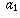,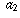,, . . . converges to infinity, then the sequence of reciprocals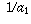,,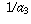, . . . converges to zero.

(Similar looking expressions like "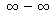" don't make any sense, for just because two sequences,,, . . . and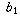,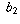,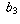, . . . each converge to infinity tells you nothing about what the sequence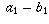,,, . . . does.)

3.  In the context of measuring sizes of sets.

The natural numbers (1, 2, 3, etc.) measure sizes of finite sets. They are possible answers to the question "how many things am I holding?"

Is there a similar concept that can measure the size of an infinite set? For instance, is there any object called "infinity" that can serve as the answer to the question "how many elements are there in the infinite set {1, 2, 3, . . . }"?

the answer is a qualified yes; however, we really shouldn't use the word "infinity" for this one object alone, because there are other infinite sets with different sizes. For more information, see the section describing the reasons for this.

For more information on the above topics, refer to one of the sections below:
• Number System Context: Why Infinity Doesn't Exist
• Topological Context: Why Infinity Does Exist
• Size of Sets Context: Why Infinity Does Exist

Navigation Panel: